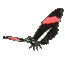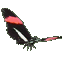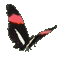Teaching Reciprocal Trig Functions - Cosecant Secant Cotangent Help - Game Tips:

- When angle A is in standard position at the graph's origin (0,0) then cosec A equals (r/y).
Since r is the hypotenuse of a right triangle, r can be found from point D(x,y) using x2 + y2 = r2 .
Consequently, cosec A can also be described as (hypotenuse)/(opposite side).
cosec(A) = h/o = r/y = reciprocal of sin(A) = 1/sin(A) .

- When angle A is in standard position at the graph's origin (0,0) then secant A equals (r/x).
Also secant A can be described as (hypotenuse)/(adjacent side).
sec(A) = h/a = r/x = reciprocal of cos(A) = 1/cos(A) .

- When angle A is in standard position at the graph's origin (0,0) then cot A equals the ratio = (x/y).
cot(A) = a/o = x/y = reciprocal of tan(A) = 1/tan(A) .

- Your Game Score is reduced by the number of butterfly hits.

- To slow the game speed repeat tap/click on the word Slider.
- To increase the game speed repeat tap/click on the word Math.
- Speed can also be adjusted with a keyboard's - and + keys.

- Refresh/Reload the web page to restart the game.